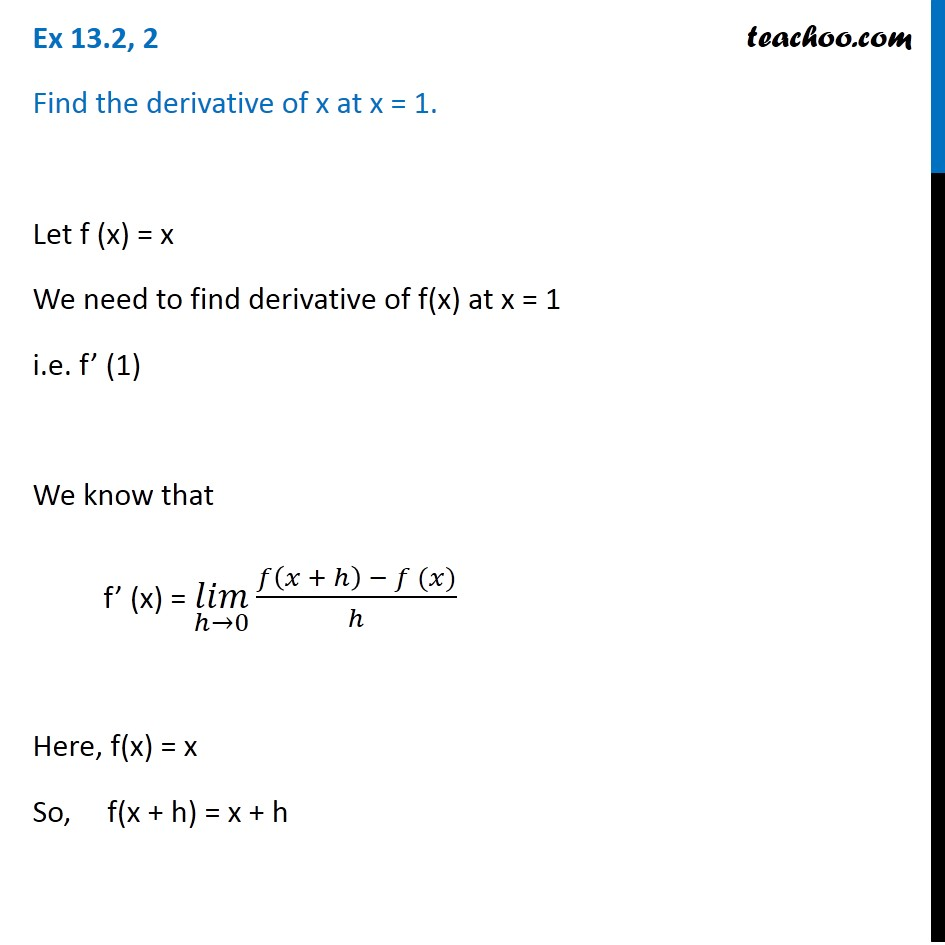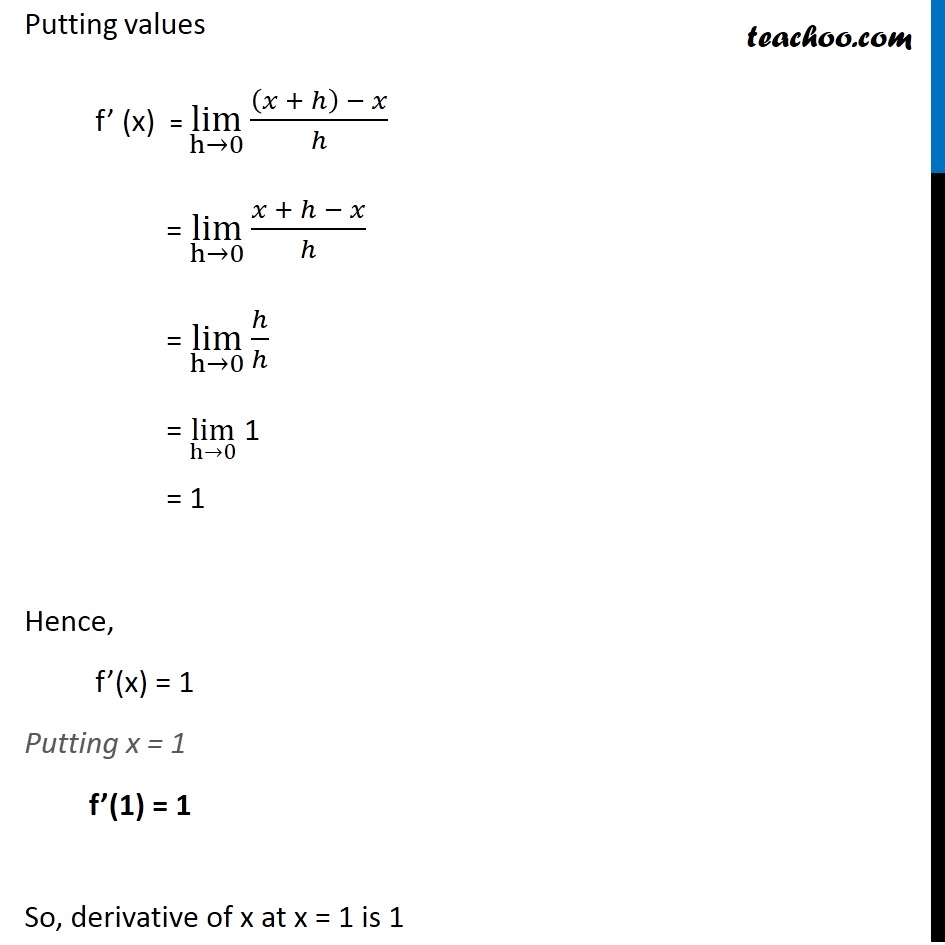1. Chapter 13 Class 11 Limits and Derivatives
2. Concept wise
3. Derivatives by 1st principle - At a point

Transcript

Ex 13.2, 2 Find the derivative of x at x = 1. Let f (x) = x We need to find derivative of f(x) at x = 1 i.e. f’ (1) We know that f’ (x) = (𝑙𝑖𝑚)┬(ℎ→0)⁡〖(𝑓(𝑥 + ℎ) − 𝑓 (𝑥))/ℎ〗 Here, f(x) = x So, f(x + h) = x + h Putting values f’ (x) = lim┬(h→0)⁡〖((𝑥 + ℎ) − 𝑥)/ℎ〗 = lim┬(h→0)⁡〖(𝑥 + ℎ − 𝑥)/ℎ〗 = lim┬(h→0)⁡〖ℎ/ℎ〗 = lim┬(h→0) 1 = 1 Hence, f’(x) = 1 Putting x = 1 f’(1) = 1 So, derivative of x at x = 1 is 1

Derivatives by 1st principle - At a point

About the AuthorDavneet Singh
Davneet Singh is a graduate from Indian Institute of Technology, Kanpur. He has been teaching from the past 10 years. He provides courses for Maths and Science at Teachoo.